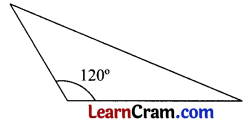# DAV Class 5 Maths Chapter 16 Worksheet 4 Solutions

The DAV Class 5 Maths Solutions and DAV Class 5 Maths Chapter 16 Worksheet 4 Solutions of Triangles offer comprehensive answers to textbook questions.

## DAV Class 5 Maths Ch 16 Worksheet 4 Solutions

Question 1.
Identify the scalene, isosceles, and equilateral triangles.
(a)Solution:
Isosceles Triangle (Two sides are equal)

(b)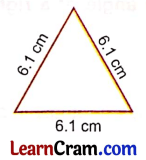Solution:
Equilateral Triangle (Three sides are equal)(c)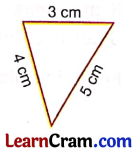Solution:
Scalene Triangle (No side are equal)

(d)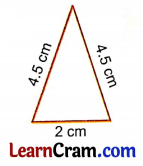Solution:
Isosceles Triangle (Two sides are equal)

(e)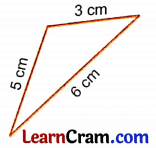Solution:
Scalene Triangle (All the sides are different)

Question 2.
Identify the acute, obtuse, and right-angled triangles.
(a)Solution:
Right-angled triangle (One angle is 90°)(b)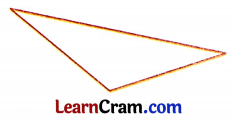Solution:
Obtuse angled triangle (One angle is obtuse)

(c)Solution:
Acute angled triangle (all angles are acute)

Question 3.
Find the third angle of this triangle. What type of triangle is this? Why?Solution:
Let the third angle be x°.
Sum of all the angles of a triangle is 180°.
So, 40° + 50° + x° = 180°
⇒ 90° + x° = 180°
⇒ x° = 180° – 90°
⇒ x° = 90°
As one angle is 90° it is a right triangle.

Question 4.
Sum of two angles of a triangle is 90°. Find the third angle. What type of triangle is this?
Solution:
Sum of two angles = 90°
Let the third angle = x°
x° + sum of two angles = 180° (sum of 3 angles of triangle is 180°)
⇒ x + 90° = 180°
⇒ x° = 180° – 90°
⇒ x° = 90°
As one angle is 90° of a triangle it is a right-angled triangle.Question 5.
If the measure of one angle of a right-angled triangle is 48°, find the other two angles.
Solution:
As it is right-angled triangle one of the angles is 90°.
Other angle = 48°
Let the third angle be x°
x° + 48° + 90°= 180°
⇒ x° + 138°= 180°
⇒ x° = 180° – 138°
⇒ x° = 42°
So, the other two angles are 90°, 42°.

Question 6.
Fill in the blanks.
(a) A triangle whose any one angle is a right angle, is called a _________ triangle.
Solution:
Right Angled

(b) Each angle of an acute-angled triangle is _________
Solution:
Acute

(c) Anyone angle of an obtuse-angled triangle is _________
Solution:
Obtuse

(d) A triangle whose any two sides are equal in length is called _________ triangle.
Solution:
Isosceles

(e) Sum of three sides of a triangle is equal to _________
Solution:
180°(f) Sum of lengths of any two sides of a triangle is _________ than the third side.
Solution:
Greater

(g) In an isosceles triangle, any _________ sides are equal in length.
Solution:
Two

DAV Class 5 Maths Chapter 16 Worksheet 4 Notes

Classification of Triangles
Triangles are classified in two ways-

• According to sides.
• According to angles.

I. Classification According to Sides
(a) If all the three sides of a triangle are of different lengths, the triangle is called a scalene triangle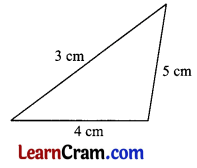(b) A triangle whose any two sides are of same length is called an isosceles triangle.(c) A triangle whose all the three sides are of equal length is called an Equilateral triangle.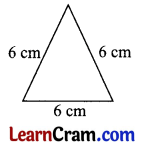II. Classification According to Angles
(a) A triangle whose all the angles are acute, is called an Acute Angled triangle.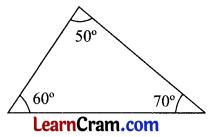(b) A triangle whose any one angle is a right angle is called a Right Angled triangle.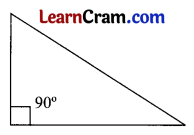(c) A triangle whose one angle is obtuse, is called an Obtuse Angled triangle.